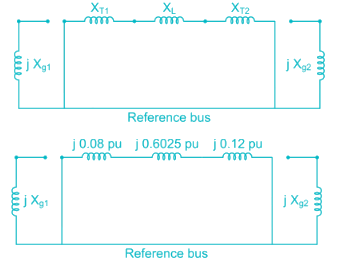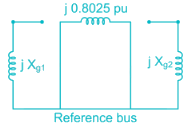Courses

# Power Systems - 6

## 10 Questions MCQ Test GATE Electrical Engineering (EE) 2022 Mock Test Series | Power Systems - 6

Description
This mock test of Power Systems - 6 for Electrical Engineering (EE) helps you for every Electrical Engineering (EE) entrance exam. This contains 10 Multiple Choice Questions for Electrical Engineering (EE) Power Systems - 6 (mcq) to study with solutions a complete question bank. The solved questions answers in this Power Systems - 6 quiz give you a good mix of easy questions and tough questions. Electrical Engineering (EE) students definitely take this Power Systems - 6 exercise for a better result in the exam. You can find other Power Systems - 6 extra questions, long questions & short questions for Electrical Engineering (EE) on EduRev as well by searching above.
QUESTION: 1

### A three phase transmission line having a self-reactance of 1 Ω/km and a mutual reactance of 0.2 Ω/km. The negative and zero sequence equivalent reactances respectively are

Solution:

Positive sequence reactance = Xs – Xm = 0.8 Ω/km

Negative sequence reactance = Xs – Xm = 0.8 Ω/km

Zero sequence reactance = Xs + 2Xm = 1.4 Ω/km

QUESTION: 2

### A balanced star connected load takes 50 A from a balanced 3-phase. 4-wire supply. The zero and positive sequence components (Iao and Ia1) of the line current (Ia) are respectively

Solution:

The given load is balanced star connected load. In balanced system only positive sequence component is present, both negative and zero sequence components are absent.

Zero sequence component, Iao = 0 A

Given that, Ia = 50A

Positive sequence component,

Ia1 = Ia = 50A

QUESTION: 3

### What is the per unit reactance of an alternator of the capacity is doubled and the voltage is halved?

Solution: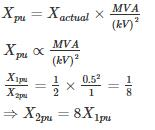*Answer can only contain numeric values
QUESTION: 4

A transmission line has an impedance of 0.3 PU on 11 KV and 200 MVA base. Find the new PU Impedance on 33 kV and 1600 MVA base

Solution:

ZPUnew = 0.3 ×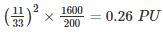QUESTION: 5

A transmission line of inductance 0.1 H and resistance 5 ohms is suddenly short-circuited at t = 0 at the bar end ass shown in the figure. The approximate value of the first current maximum (Maximum momentary current) is

Solution:

Z = 5 + j 314 × 0.1 = 5 + j 31.4 = 31.8 ∠81°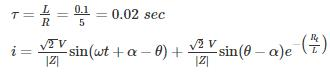= 3.14 sin (314 t - 66) + 2.87 e-50 t

First current maximum of symmetrical short circuit current occurs at

57.3 × 314 t - 66 = 90°

⇒ t = 0.00867 sec

First current maximum

imm = 3.14 + 2.87 e-50 × 0.00867 = 5 A

*Answer can only contain numeric values
QUESTION: 6

Two power stations S1 and S2 are interconnected through a transmission line of per unit (p.u) reactance of 0.6. Station S1 has one generator of P.U. the reactance of 0.3, and station S2 has two generators of p.u reactance 0.6 each. For a 3 – phase symmetrical short circuit at the middle of the transmission line (point F). The change in the value of the equivalent fault reactance, with one of the generators of S2 removed, is _____ (in %).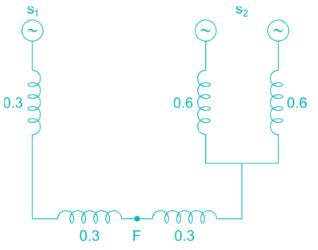Solution:

Equivalent reactance with respect to fault point =(0.3 + 0.3) || (0.3 + (0.6 || 0.6))

=0.6 || (0.3 + 0.3)

= 0.3

After removal of one of the generators of S2 with respect to fault point

= (0.3 + 0.3) || (0.3 + 0.6)

= (0.6) || (0.9) = 0.36
Change in the reactance == 20%

*Answer can only contain numeric values
QUESTION: 7

For a generator, the ratio of fault current for Line to Line fault and three phase fault is 0.5.

The positive sequence reactance is 0.2 μ. The negative sequence reactance is __(in p.u.)

Solution: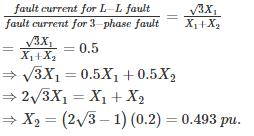QUESTION: 8

A three-phase Y-Δ transformer is rated 400 MVA, 220 kV/22 kV. The short circuit impedance of the transformer measured on low voltage side is 0.121 Ω and because of the low value, it is considered equal to the leakage reactance. The per unit value of reactance to represent this transformer in a system whose base on the high voltage side of the transformer is 100 MVA, 230 kV is ___ (pu)

Solution:

On its own base the pu reactance of the transformer is,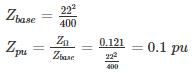On the chosen base the reactance becomes,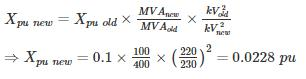*Answer can only contain numeric values
QUESTION: 9

A 3ϕ load is connected to a 3ϕ balanced supply Van = 20∠120°, Vbn = 20∠-120°, Vcn = 20∠0° find the value of R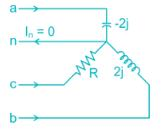Solution:

Apply KCL
Ia + Ib + Ic = In = 0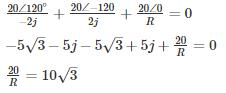R = 1.154 Ω

QUESTION: 10

Two 300 MVA, 22 kV, 3-phase, 60 Hz alternators are connected by a transmission line having transformers at both ends as shown in the single line diagram given below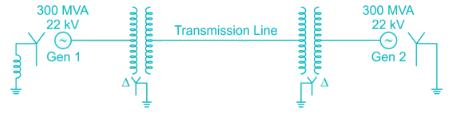The transformer (T1) between the generator 1 and the transmission line is a Δ-Y connected 3-phase transformer bank made up of three single phase transformers each rated at 120 MVA, 22 kV/127 kV with leakage reactance of 8%. The generator 2 is connected to the line by a 3-phase, 300 MVA, 22 kV/220 kV, Δ-Y transformer (T2) with 10% leakage reactance. The star side of T1 and T2 are grounded. The zero sequence reactance of the transmission line is 81 Ω. Considering the MVA rating of T1 as the base, the zero-sequence network of the complete system is (Assume Xg1 and Xg2 are the zero sequence reactance of the generators respectively)

Solution:

Given that base MVA is the rating of T1

Rating of single phase transformer of 3-phase transformer bank = 12 MVA

Rating of 3-phase transformer bank = 3 × 120 = 360 MVA

It is connected in Δ Y connection.

Primary voltage of three phase transformer bank = Primary voltage of single phase transformer = 22 kV

Secondary voltage of three phase transformer bank = √3 × secondary voltage of single phase transformer = √3 × 127kV = 220kV

XT1 (for single phase transformer) = j0.08

XT1 = (for three phase transformer)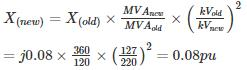XT1 = j0.08 pu

XT2 (old) = j0.10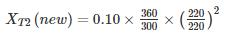= j 0.12 pu

Given that, reactance of transmission line (XL) = 81Ω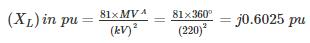As the generator reactance values are not given, let assume zero sequence reactance of generator 1 and generator 2 are Xg1 and Xg2 respectively.

Now, the zero-sequence network becomes.